## Pileup

Last updated October 7, 1998

• Table of pileup fractions for all tests
• Memo in Postscript format: HEXS Flat Field Pileup Analysis, Stephen Jones, 8/2/97.
• Memo in Postscript format: An Analytical ACIS Pileup Model, Brian McNamara, 10/2/97.
• Memo in Postscript format: ACIS Pileup: Analysis of XRCF Phase H Data, Joel Kastner, Kester Allen, & Kenny Glotfelty, 2/24/98.

• ## 1. MONOCHROMATIC PILEUP VS. A SIMPLE PILEUP MODEL

Figure 1 shows the correlation between the photon pileup fraction and the number of detected photons per CCD frame. The data were extracted from 15 on-axis, focussed, point spread function and count rate linearity measurements obtained at the XRCF during Phase H testing. The x axis shows the number of incident X-ray photons per ACIS frame, calculated as

fBND × EA (HRMA + ACIS) × tf ;

fBND is the mean X-ray flux (photons/sec/cm2) at the HRMA entrance aperture, EA (HRMA + ACIS) is the current baseline model for the product of the HRMA effective area × ACIS QE × optical blocking filter transmission, and tf is the ACIS frame time. The y axis shows the degree of pileup determined using two methods. The diamonds represent the ratio of the sum of photons in the n = 2-3 or n = 2-4 spectral pileup peaks to the total number of photons in the main peak (n=1) + the pileup peaks, calculated as

pp= sumn > 1 [An × n] / sumn >= 1 [An × n],

where An is the area under the main peak or pileup peak.

Figure 1.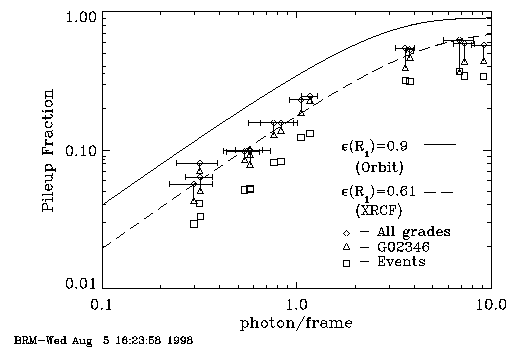• This plot is also available in Postscript format.

The degree of photon pileup was determined for all grades (diamonds) and for ASCA grades 02346 (connected triangles). The data, which range in energy between 1.486 keV and 6.4 keV, were obtained with both the front-illuminated and back-illuminated CCDs. In addition, we calculated the degree of event pileup as

pe = sumn > 1 [An] / sumn >=1 [An].

These data are plotted as squares in Fig. 1, and are tabulated in Table 1. The degree of event pileup is roughly a factor of two smaller than the degree of photon pileup.

The data are compared in Fig. 1 to an analytic ACIS pileup model (see the memo entitled ``An Analytical ACIS Pileup Model'' 1997, by B.R. McNamara). The solid and dashed lines were produced using the McNamara (1997) pileup model. The model has one free parameter: the encircled energy (detected photons) within the central pixel island detection cell in the limit of zero pileup, approximately 2.1 pixels.

In order to scale the model to the data, we measured the encircled energy fraction within 2.1 pixels for the three tests with the lowest degree of pileup in our study (H-IAS-PI-1.041, H-IAI-CR-1.001, H-IAS-CR-1.002). The photon pileup fractions for these tests are less than 8%. It is necessary to scale the model using the encircled energy radius in the limit of zero pileup because, the measured encircled energy radius migrates outward as the degree of pileup increases (see below). The mean encircled energy within the central detection cell (2.1 pix) for these tests was found to be epsilon(r)=61%. The scaled model (dashed line) fits the all grade (diamonds; appropriate for the model comparison) photon pileup fraction quite well over the full range of the data. The degree of photon pileup is underestimated when using G02346 data because of grade migration.

The model systematically overestimates the degree of event pileup, unless epsilon(r) <<61%, which is inconsistent with the data.

This simple pileup model is able to predict reasonably well the degree of pileup in XRCF data spanning a broad range of energy and pileup conditions, for both front and back illuminated CCDs. The solid line shows the degree of pileup expected for an on-axis point source on orbit (minus gravity, finite distance, and finite source size). The degree of pileup for a given number of counts per frame will be ~2× larger on orbit because of the sharper PSF.

## 2. PILEUP SIGNATURES IN FOCUSSED IMAGES

In order to correct for pileup in flight science data, the observer will need some understanding of the source flux (degree of pileup) and the shape of the unpiled spectrum. These are in general unknown quantities. We therefore need some independent way of estimating the degree of pileup that does not require that the spectrum and flux be known.

We have found at least three characteristics of the focussed point spread function that are sensitive to the degree of pileup: the encircled energy radius, the slope of the point spread function in the core as a function of event grade, and the integrated area of the core of the point spread function (PSF) as a function of event grade.

The change in the 50%, 70%, and 90% encircled energy radius as a function of the detected number of photons per frame for the tests shown in Fig. 1 is shown in Fig. 2. Fig. 2 shows the increase in the encircled energy radius with increasing flux. This trend is due to decrease in the number of detected events in the core of the PSF relative to the wings of the PSF caused by pileup in the core. The trend is most obvious and has the least scatter when plotting the 50% encircled energy radius, which is most sensitive to the number of detected events in the core of the PSF.

Figure 2.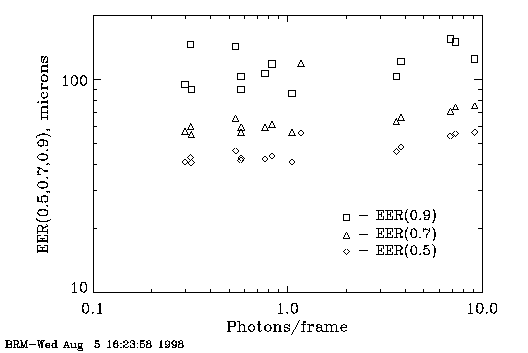• This plot is also available in Postscript format.

We now ask how sensitive is the encircled energy radius to the degree of pileup for purposes of estimating the degree of pileup without knowledge of the incident flux. In Fig. 3 we plot the photon pileup fraction against the 50% encircled energy radius (EER(0.5)) on the left axis, and we compare this to the photon pileup fraction plotted against the number of photons per CCD frame on the right axis (i.e. Fig. 1). The solid curve is the pileup model fit to the photon pileup fraction as a function of the number of photons per frame (Fig. 1), and the dashed curve is a second order polynomial fit to the EER(0.5) vs the photon pileup fraction. This plot shows that the EER(0.5) is relatively insensitive to the degree of pileup for pileup fractions less than 40%, or about 2 photons per frame. The sensitivity of EER(0.5) increases sharply as the degree of pileup increases above 40%.

Figure 3.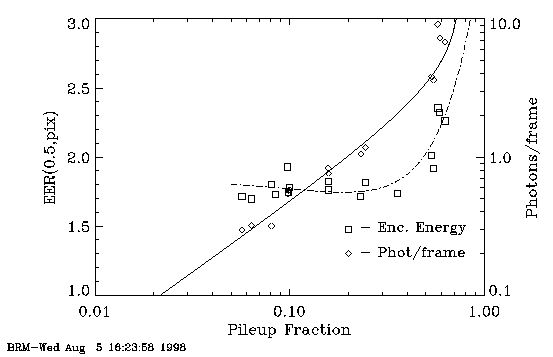• This plot is also available in Postscript format.

Most science observations should be planned so that the degree of pileup is considerably less than 40%, and we expect most to be in the few to 10% range. We therefore investigated more sensitive measures of pileup. In Fig. 4 we plot the change in the integrated area of the PSF profile A(G) between G02346 and all grades, extracted from 2 pixel-wide slices (Y-direction) for each focussed image. Inspection of these data for each test show that the area under the G02346 PSF decreases with respect to the PSF for all grades as pileup increases (See Table X and associated data). This is the result of grade migration. Similarly in Fig. 5 we plot the change in the peak amplitude of the PSF between all grades and G02346. We plot the pileup model and data (right axis) in Figs. 4 & 5 as we did in Fig. 3. The dashed curve in Fig. 4 is a third order polynomial fit to A(G) vs pileup fraction (squares), and the dashed curves in Fig. 5 are two linear fits to P(G) vs pileup fraction. The first is fit to the range Pp=0.05-0.4 and the second fits the range Pp=0.4-1.0.

Figure 4.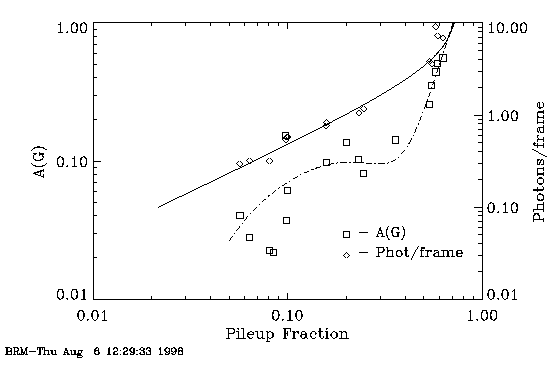• This plot is also available in Postscript format.

We note the following from these figures:

• both P(G) and A(G) are more sensitive to the degree of pileup for Pp=0.05-0.4 than is EE(0.5). However the trend with Pp is weak in this range and there is considerable scatter. In general, P(G)~A(G)~0.5 × Pp.

• the slopes in the trends between P(G) and A(G) vs Pp increase sharply at Pp=0.4, where P(G)~A(G)~Pp
Figure 5.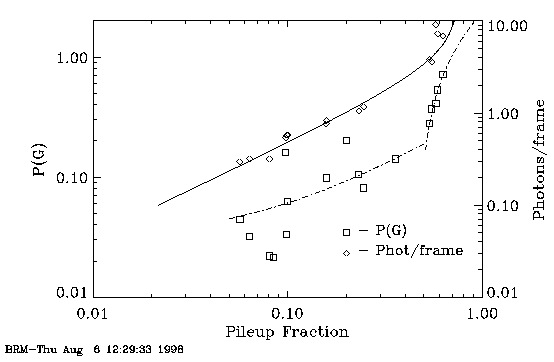• This plot is also available in Postscript format.

## 3. CONCLUSIONS

1) The in-focus, on-axis, monochromatic PSF images show a well defined trend between pileup fraction (photon and event) and the number of photons per CCD frame determined using BND measurements and the AXAF-ACIS effective area model.

2) The one parameter, analytic pileup model proposed by McNamara predicts the photon pileup fraction as a function of the number of detected photons per frame to within several percent. However, the model systematically overpredicts the event pileup fraction as a function of the number of detected photons per frame by ~2×.

3) We have shown that the structure of the PSF as a function of photon event grade can be used to estimate the degree of pileup for nearly monochromatic, focussed, on-axis data. However, the sensitivity of the PSF structure to pileup is weak for pileup fractions below ~40%. A spectrum-independent means of estimating the degree of pileup in science observations will be important when attempting to model and remove pileup from the observed spectra. The sensitivity of the PSF structure to pileup may increase for modest pileup levels with the sharper, on-orbit PSF.

— B.R. McNamara, A. Ware